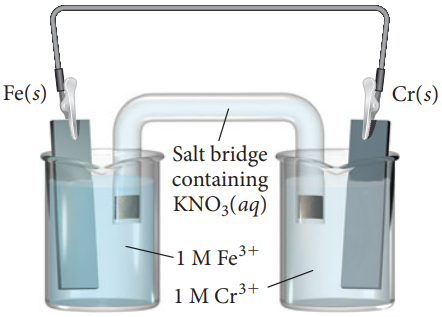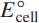×
Get Full Access to Chemistry: A Molecular Approach - 3 Edition - Chapter 18 - Problem 47e
Get Full Access to Chemistry: A Molecular Approach - 3 Edition - Chapter 18 - Problem 47e

×

# Consider the voltaic cell: a. Determine the direction ofISBN: 9780321809247 1

## Solution for problem 47E Chapter 18

Chemistry: A Molecular Approach | 3rd Edition

• Textbook Solutions
• 2901 Step-by-step solutions solved by professors and subject experts
• Get 24/7 help from StudySoup virtual teaching assistantsChemistry: A Molecular Approach | 3rd Edition

4 5 1 420 Reviews
25
3
Problem 47E

Consider the voltaic cell:a. Determine the direction of electron flow and label the anode and the cathode.

b. Write a balanced equation for the overall reaction and calculate.

c. Label each electrode as negative or positive.

d. Indicate the direction of anion and cation flow in the salt bridge.

Step-by-Step Solution:

Problem 47E

Consider the voltaic cell:a. Determine the direction of electron flow and label the anode and the cathode.

b. Write a balanced equation for the overall reaction and calculate.

c. Label each electrode as negative or positive.

d. Indicate the direction of anion and cation flow in the salt bridge.Step by Step Solution

Step 1 of 3

A voltaic cell is an electrochemical cell with two electrodes. The electrode which undergoes oxidation is called anode and the one which undergoes reduction is called cathode.

For a pair of electrodes, we can determine which one is anode and which is cathode by knowing their standard reduction potentials. Which have the least positive or highest negative potential undergo oxidation. whereas the one with least negative or highest positive potential undergoes reduction and acts as cathode.

Step 2 of 3

Step 3 of 3

##### ISBN: 9780321809247

Chemistry: A Molecular Approach was written by and is associated to the ISBN: 9780321809247. The answer to “?Consider the voltaic cell: a. Determine the direction of electron flow and label the anode and the cathode. b. Write a balanced equation for the overall reaction and calculate . c. Label each electrode as negative or positive. d. Indicate the direction of anion and cation flow in the salt bridge.” is broken down into a number of easy to follow steps, and 51 words. This textbook survival guide was created for the textbook: Chemistry: A Molecular Approach, edition: 3. The full step-by-step solution to problem: 47E from chapter: 18 was answered by , our top Chemistry solution expert on 02/22/17, 04:35PM. This full solution covers the following key subjects: direction, label, flow, electron, calculate. This expansive textbook survival guide covers 82 chapters, and 9454 solutions. Since the solution to 47E from 18 chapter was answered, more than 280 students have viewed the full step-by-step answer.

Unlock Textbook Solution